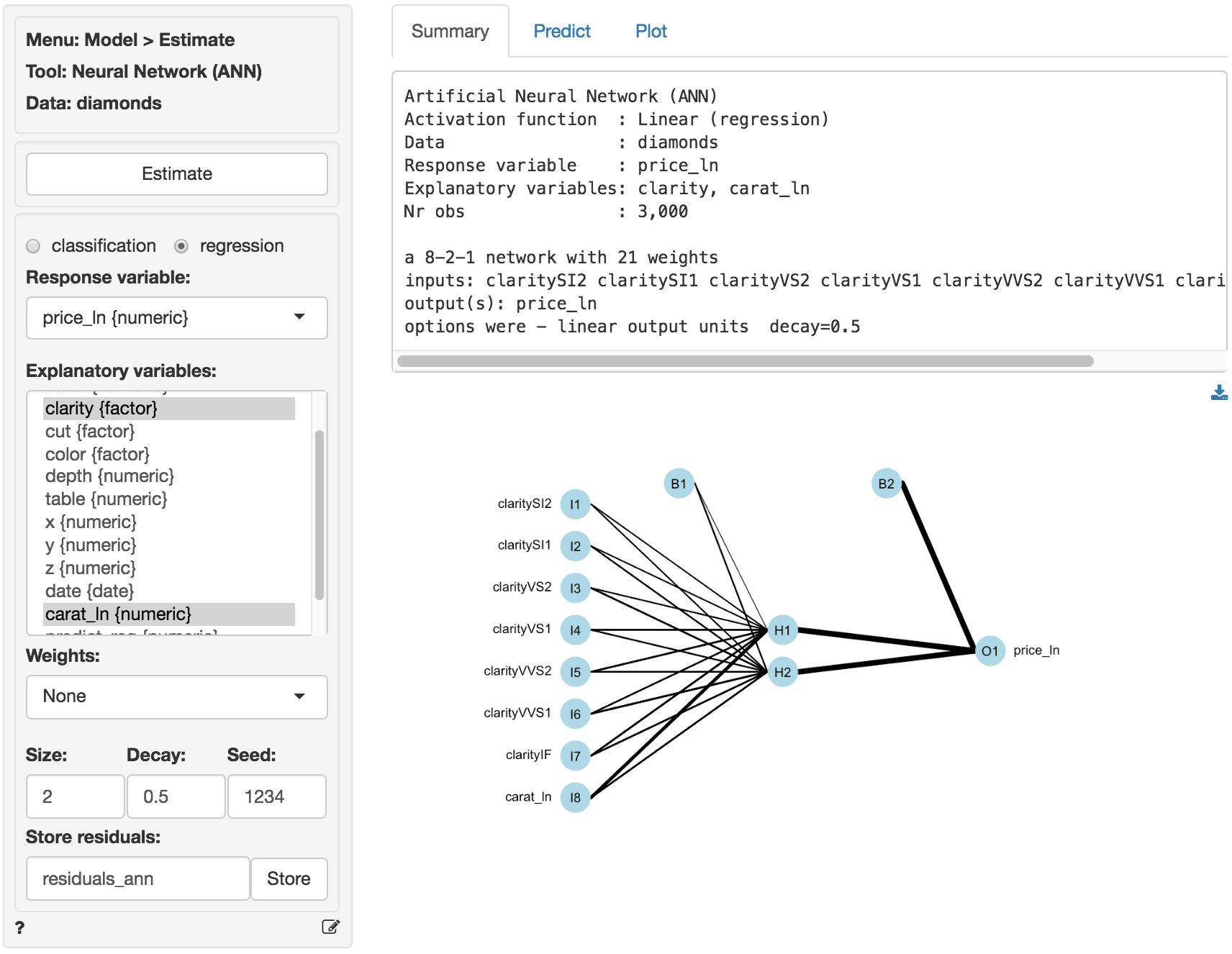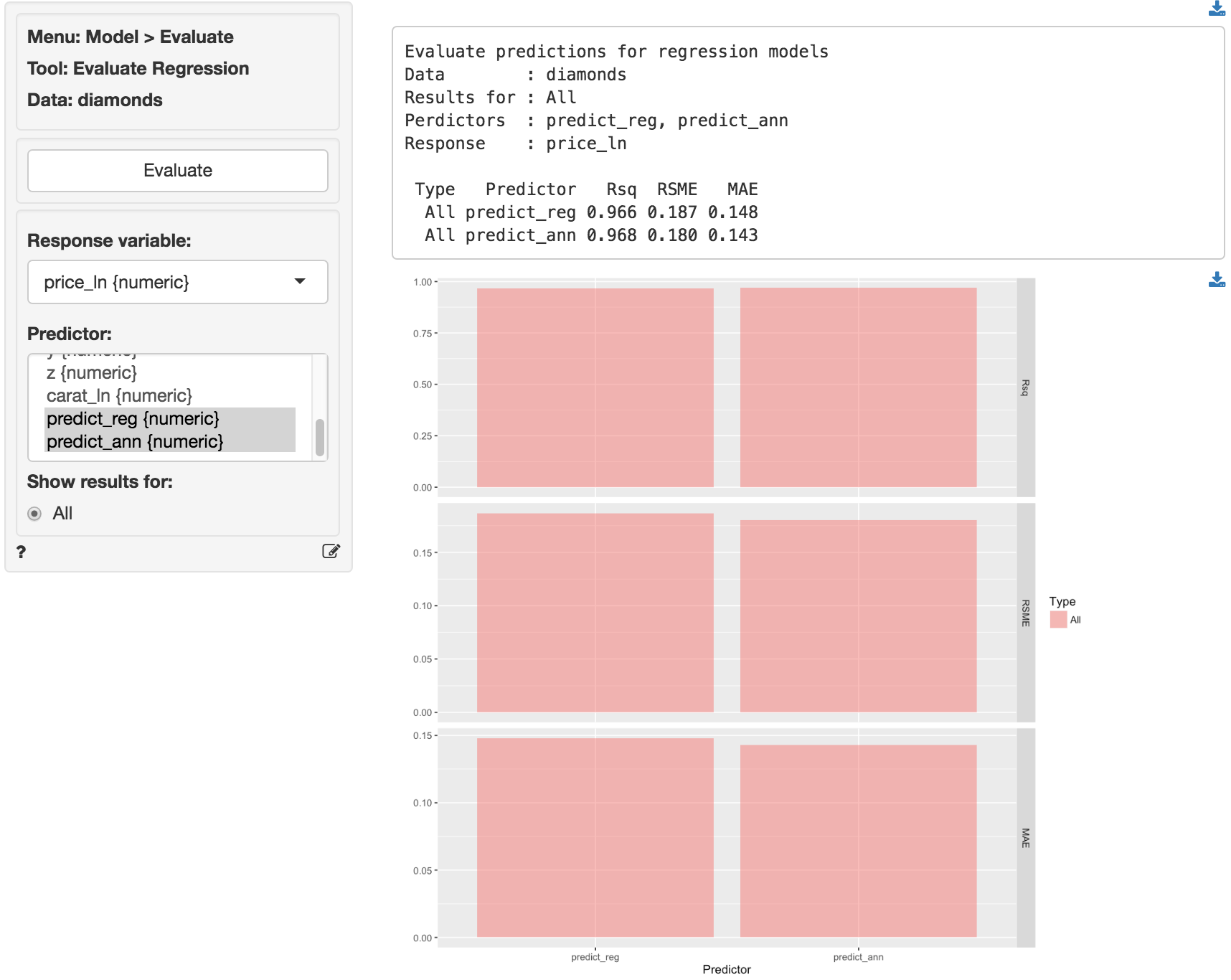Evaluate regression model performance

To download the table as a csv-files click the top download button on the right of your screen. To download the plots at a png file click the lower download icon on the right of your screen.

#### Response variable

The numeric outcome, or response, variable of interest.

#### Predictor

Select one or more variables that can be used to predict the value of the response variable. This could be a variable or predicted values from a model (e.g., from a regression estimated using Model > Linear regression (OLS) or a Neural Network estimated using Model > Neural Network (ANN)).

#### Show results for

If a filter is active (e.g., set in the Data > View tab) generate results for All data, Training data, Validation data, or Both training and validation data. If no filter is active calculations are applied to all data.

## Example

Predictions were derived from a linear regression and an neural network with two nodes in the hidden layer on the diamonds data. The variables price and carat were log-transformed prior to estimation.The data is available through the Data > Manage tab (i.e., choose Examples from the Load data of type drop-down and press Load examples). The predictions shown below were generated in the Predict tab.The test statistics show a small, but consistent, advantage for the ANN.© Vincent Nijs (2016)